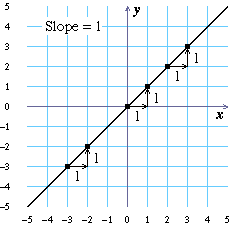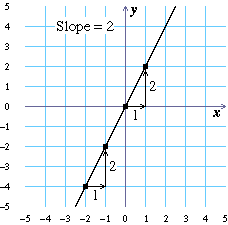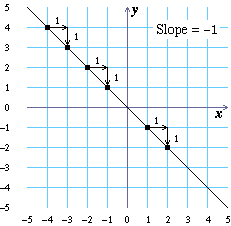# Investigating \$y=mx\$

The slope of a line is a number that measures how steep the line is. In this lesson you will learn about the meaning of slope, and find the slopes of several linear graphs.

## Lines with positive slope

If \$y=2x\$, what will \$y\$ be if \$x=5\$? Enter this value in the \$y\$ column corresponding to \$x=5\$ in the table to the right. Notice that a blue point is added to the grid to the left, with coordinates given by the row you just completed. Repeat this for the other \$x\$ values in the table, using the equation \$y=2x\$. (The equation \$y_1=x\$, which you learned about in the last lesson, is already graphed on the grid.)
\$x\$\$y\$
 What shape do you think the graph of \$y=2x\$ will be (triangle, circle, line, square, …)?
 Which points are higher when \$x\$ is positive, the red points from \$y_1=x\$ or the blue points from \$y=2x\$?

Click to graph the equation \$y=2x\$ on the grid to the left.

 Does the graph of \$y=2x\$ look like you thought it would?
 Think about what the graph of the equation \$y=3x\$ would look like if it were placed on the grid with these two lines. When \$x\$ is positive, would it be higher than both lines, lower than both lines, or between the two lines?
 The slider for \$m\$ is set to 3 in the equation \$y=mx\$, so the equation for the green line is \$y=3x\$. Does the graph of \$y=3x\$ appear as you guessed it would?

Use the slider in the lower left portion of your screen to change the value of \$m\$ and answer the following questions.

 Look at the graph of \$y=mx\$ as you slide \$m\$ from 0 to 5. Does the graph become steeper or less steep?
 Look at the graph of \$y=mx\$ as \$m\$ decreases from 5 to 0. Does it become steeper or less steep?
 When \$m=0\$, is the graph of \$y=mx\$ horizontal, vertical, or neither?
 What value does \$m\$ need to have for the graph of \$y\$ to match the graph of \$y_1=x\$?
 What value does \$m\$ need to have for the graph of \$y\$ to match the graph of \$y_2=2x\$?

When you slide \$m\$ the graph of \$y=mx\$ changes in steepness. The measure of this steepness is called the slope of the line.The slope of a line is the amount of change in the height of the line each time you go 1 unit to the right.

Use the slider and the graphs to the left to complete the table to the right. The equation for \$y\$ is shown below the grid to the left. Calculate each slope by looking at the graph.
equation\$m\$slope
 What do you think the slope of the line is when \$m=10\$?

## Lines with negative slope

If \$y_1=-x\$, what will \$y_1\$ be if \$x=5\$? Enter this value in the \$y_1\$ column corresponding to \$x=5\$ in the table to the right. Repeat this for the other \$x\$ values in the table, using the equation \$y_1=-x\$.
\$x\$\$y_1\$
 Think about the graph of \$y_1=-x\$. Will it have the same shape as the graphs from earlier in the lesson, or a different shape?
 Earlier in this lesson, as \$x\$ moved to the right, the plotted values moved up. As \$x\$ moves to the right on this graph, do the \$y_1\$-values move up or down?
Complete the table of values for \$y_2=-2x\$:
\$x\$\$y_2\$
 Will the graph of \$y_2=-2x\$ have the same shape as the graphs from earlier in the lesson, or a different shape?
 Which points are higher when \$x\$ is positive, the blue points from \$y_2=-2x\$ or the red points from \$y_1=-x\$?

The equations \$y_1=-x\$ and \$y_2=-2x\$ are graphed on the grid to the left.

 If you graphed \$y=-3x\$, would it have the same shape as the graphs from earlier in the lesson, or a different shape?
 When \$x\$ is positive, would the graph of \$y=-3x\$ be higher than the graphs of \$y_1=-x\$ and \$y_2=-2x\$, lower than the graphs of \$y_1=-x\$ and \$y_2=-2x\$, or between the graphs of \$y_1=-x\$ and \$y_2=-2x\$?
 Give an equation for a line with a graph that would fall between the red and the blue lines. (Hint: In \$y=mx\$, what could \$m\$ be?)

The line shown below has slope \$-1\$ because as the \$x\$-coordinate is increased by 1 you must move down 1 (in the \$-1\$ direction) to return to the line.## Putting it all together

 What happens to the graph of \$y=mx\$ as you slide \$m\$ from 0 to \$-5\$? Does it become steeper or less steep?
 What happens to the graph of \$y\$ as \$m\$ goes from 0 to 5? Does it become steeper or less steep?
 What value does \$m\$ need to have for the graph of \$y\$ to match the graph of \$y_1=x\$ (the red line)?
 What value does \$m\$ need to have for the graph of \$y\$ to match the graph of \$y_2=-x\$ (the blue line)?

Use the slider and the graph to the left to complete the table below. Use the grid to determine the slope of the green line.

equation\$m\$slope
 What do you think the slope of \$\$y={1}/{5}x\$\$ is?

You don’t need to see the graph of an equation to find its slope. Instead, you can just use the equation to determine how much \$y\$ changes when you increase \$x\$ by 1.

In each row of the table, find the slope of \$y=2x\$ by subtracting its \$y\$-values at the two indicated points, where \$x\$ increases by 1. The line, and your two points, will be plotted on the grid to the left.

\$x\$- and \$y\$-
values
slope
computation

Notice that you can calculate the slope starting anywhere on the line, and you’ll get the same answer.

Because you get the same slope for a straight line wherever you start from, you might as well pick simple \$x\$-values to use. The simplest \$x\$-values are almost always \$x=0\$ and \$x=1\$.

Find the slopes of these lines by subtracting their \$y\$-values when \$x=0\$ and \$x=1\$.

equation for
the line
\$x\$- and \$y\$-
values
slope
computation

As you can see from the last few questions:

The slope of the line \$y=mx\$ is \$m\$.